Here's a talk on "Math And Analogies" I did for the Center on Contemporary Art (CoCA) in Seattle.

Transcript: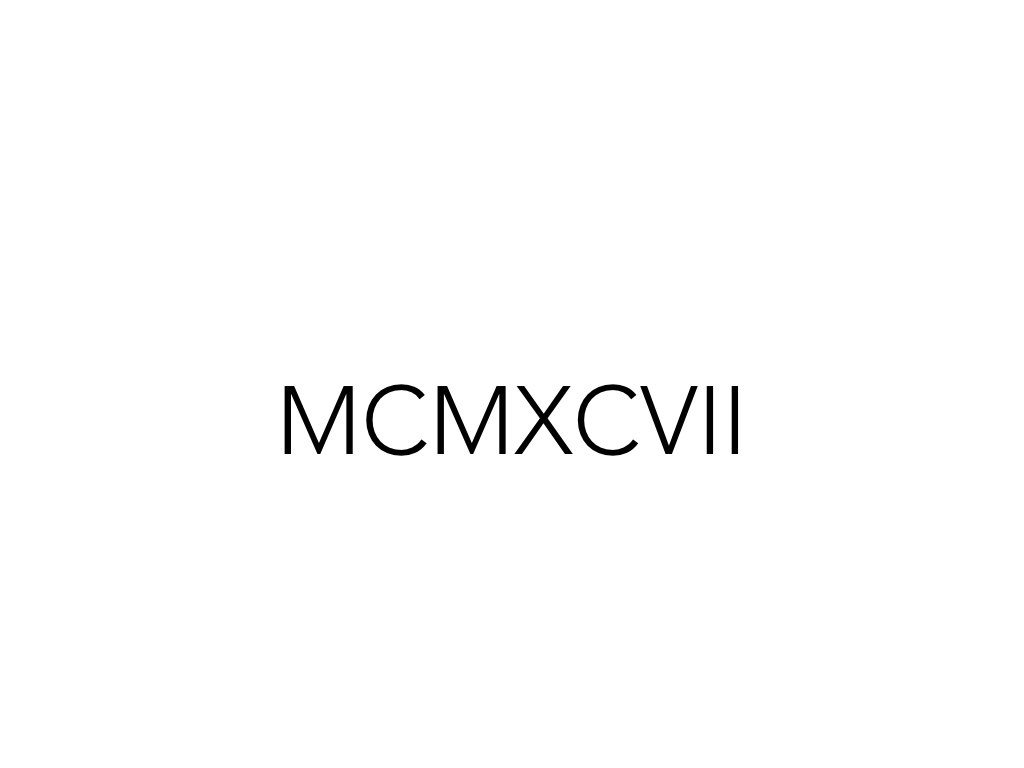What's this? Well, it's a number. Probably written down a few thousand years ago. Great. And people, I suppose, could have read it back then if they spent enough time. And if we want, we can figure it out by spending time on it too (sigh).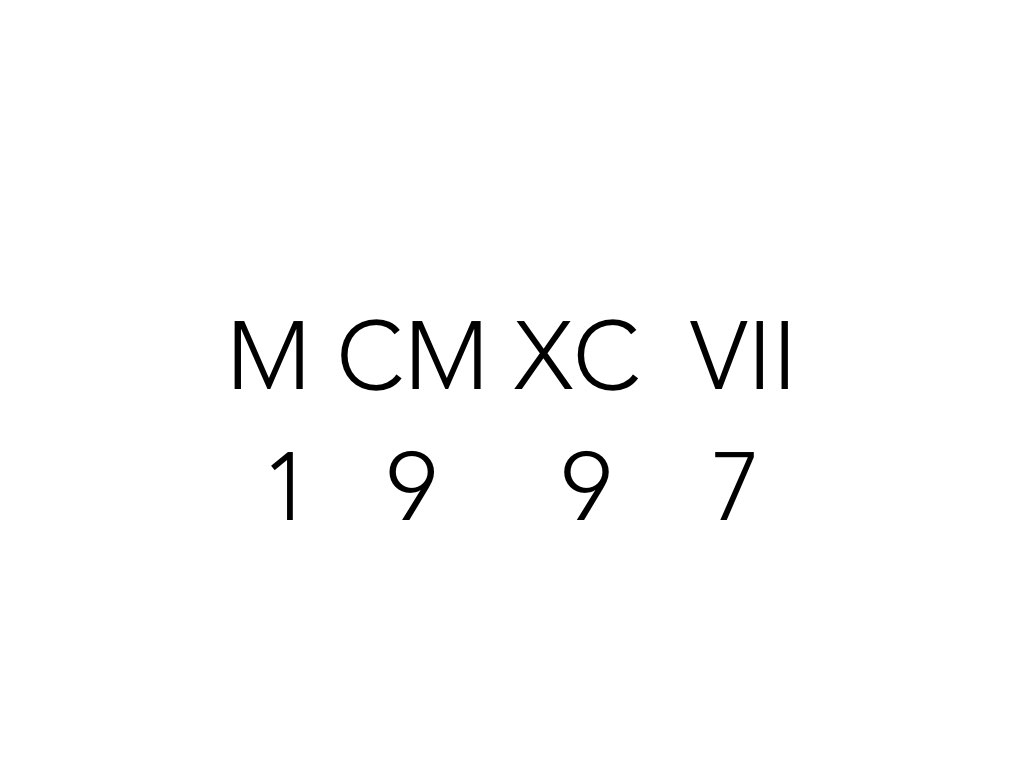So let's see. We have M, that's 1000. Then we have CM, that's 100 less than 1000, or 900. Then we have XC, which is 10 less than 100, or 90. Then we have VII, which is two more than 5, or 7. So the number is 1997.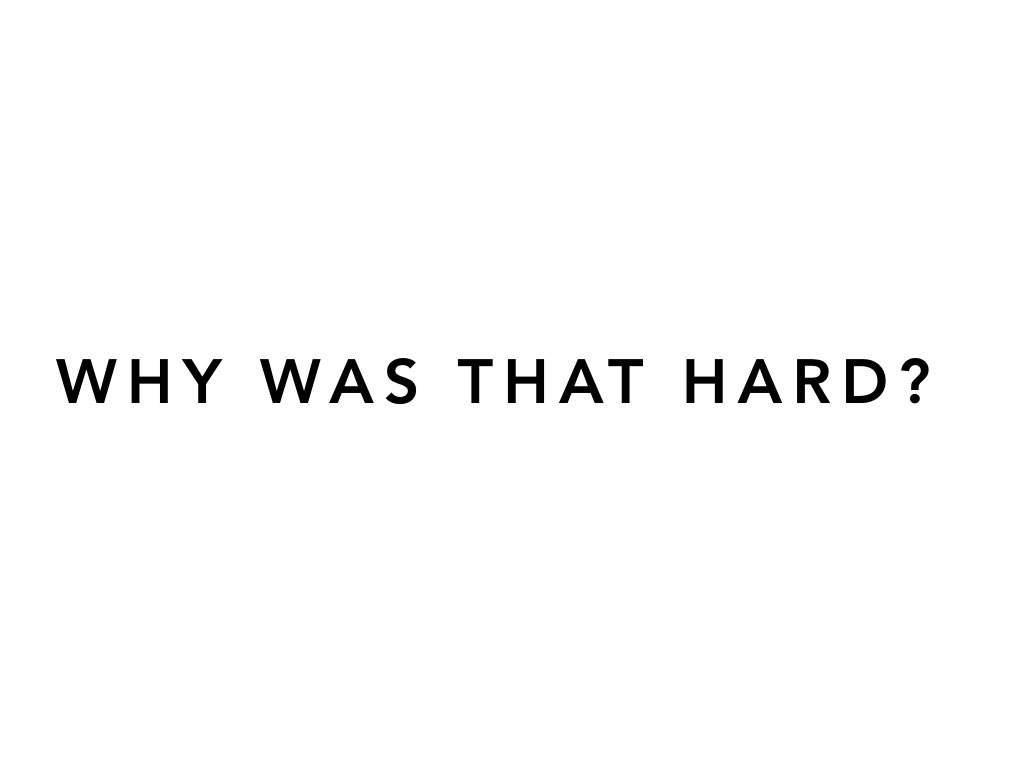OK, that wasn't fun. The question: why was that hard? Why was that difficult? Why was one representation so much easier? Well, there's a few answers.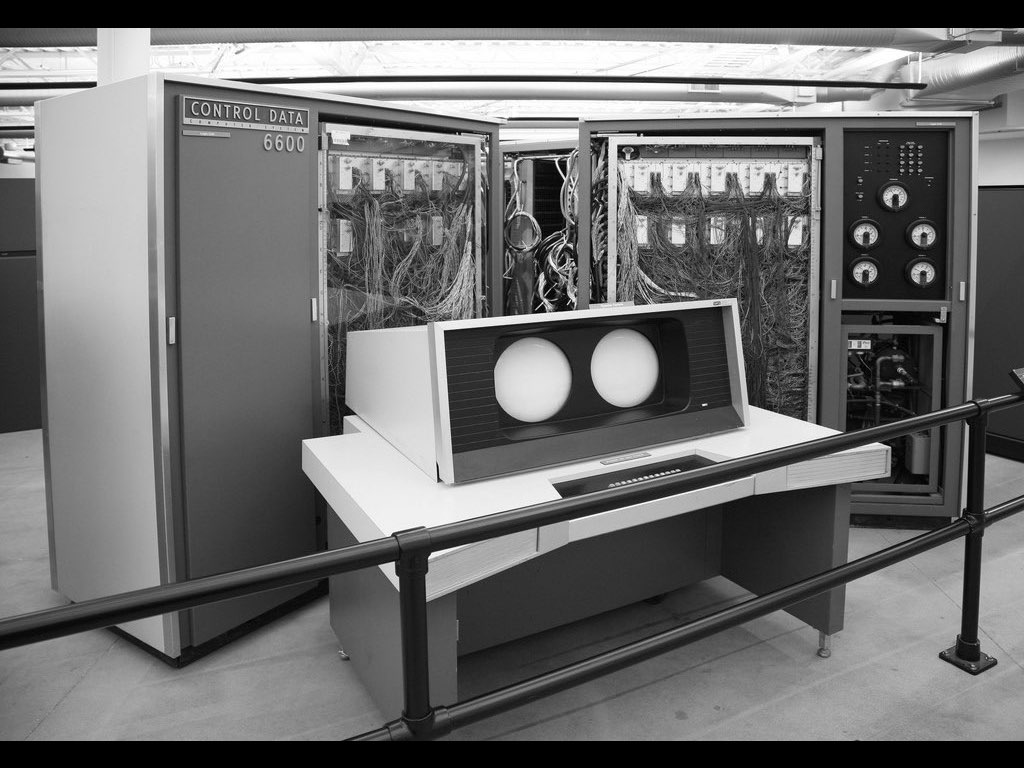One is that hey, we're just not smart enough.

Sorry guys, our brains just can't handle Roman numerals. I mean, computers can use them, why can't we? We're just not smart enough. And we're just bad at math.

That's one answer, and maybe it's possible that humans are incapable of understanding numbers.But I think the better answer is that those numbers were poorly designed. Roman numerals aren't a great representation of numbers. We're trying to keep track of numbers in terms of two more than five, and one less than ten. We can't just say 9, we need to say "one less than ten", and so on. It makes things really complicated and there's not really a good reason for it. The result is that it's cumbersome, hard to use, and our brains are doing extra work they don't have to.My goal for learning is to find the analogies that help things make sense. The analogy of numbers being in terms of "two more than five", "one less than ten" isn't a great system. Decimals are much better and that's what we use today.

The idea is that an analogy is like a raft you use to cross a river. The river is the problem, the concept, something that you want to understand, and the raft is how you approach it.

If you don't have an analogy at all, yes, maybe you're strong enough to swim to the other side by pure brute force, but usually you have a mental model that helps you with the problem. The problem is some mental models aren't as good as others. The idea of Roman numerals, while it "works", is not as good as decimals. We're taking a weaker raft to cross the river.

Let's take a look at a few mental models we've tried so far.Rocks were the first mental model for counting. The word calculus (calculate) comes from "pebble", and the idea that numbers are basically rocks being counted is a powerful analogy. It sounds simple, but it's powerful.

The reason is we've taken out all the differences. There's a bunch of rocks in this photo. Different sizes, colors, weights, everything -- but we're counting them as rocks. And that's the big idea: we take things that are different in countless ways, but find the unifying principle behind them. And now we're counting that.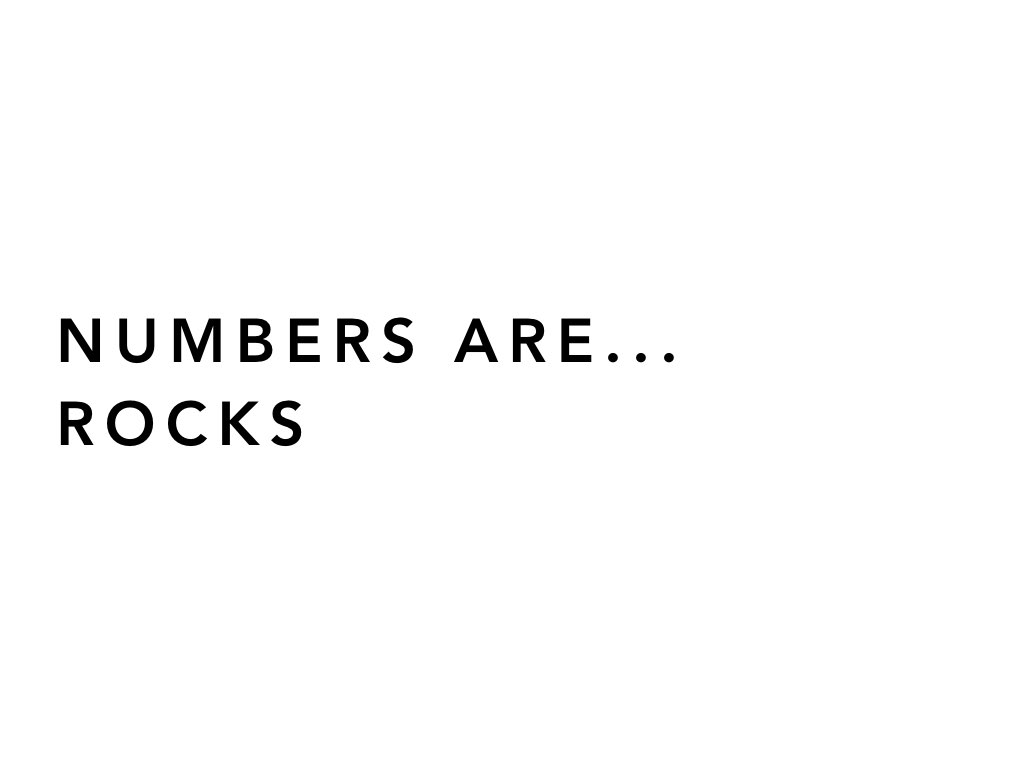So the first concept (mental model) is that numbers are like rocks. Numbers are these physical things that unify disparate items and we can count them together and it works pretty well. One, two, three, four, five, six, seven, eight, nine, ten. With enough time we can count a herd of sheep. We can count money, it works pretty well.But what's this?

Well, it's zero. Zero rocks, zero sheep, zero billion dollars are right there. Zero of the most beautiful painting in the world is right there.

Zero is a really weird concept. And when you've tied your concept of a number to physical items, concepts like zero become difficult.

Now we might work through it and say, "OK, let's allow for nothingness". So we can have "nothingness" as well as "somethingness".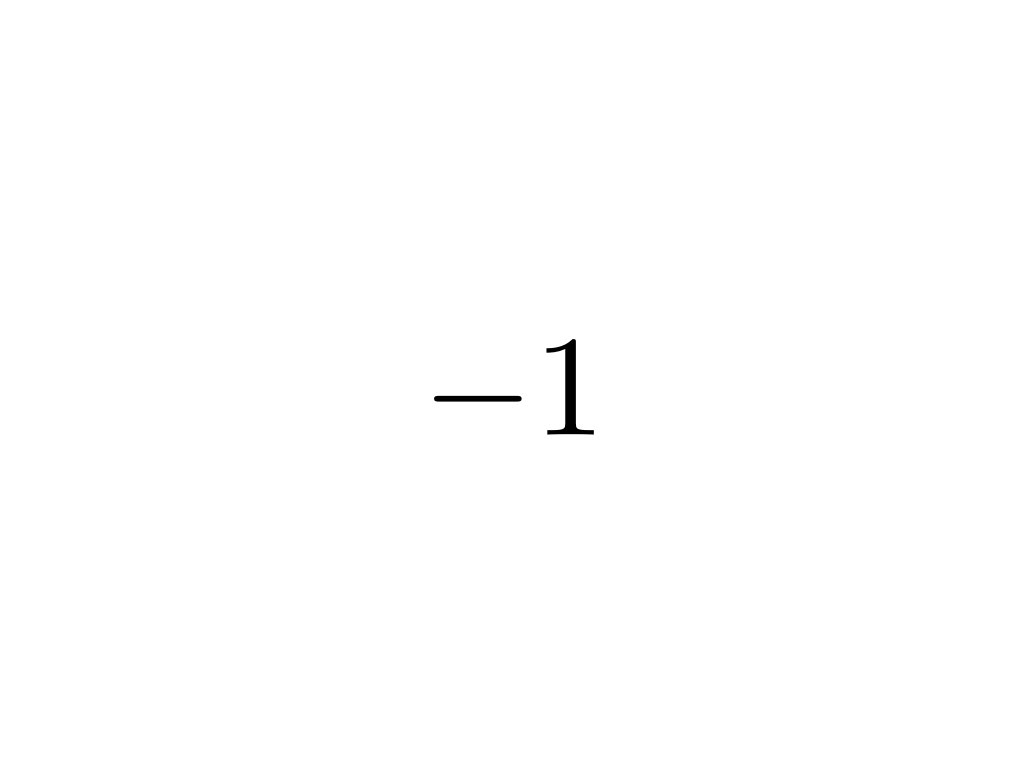Is it a rock that you owe? Well, if you're holding it, it's not negative, right? Is negative money something I owe you? Again, if I gave it to you, it might be negative to me, but it sure seems positive to you. There's a lot of things that get messed up when we're trying to use a very physical analogy. So the analogy, the raft is starting to break down. It's not helping us understand things.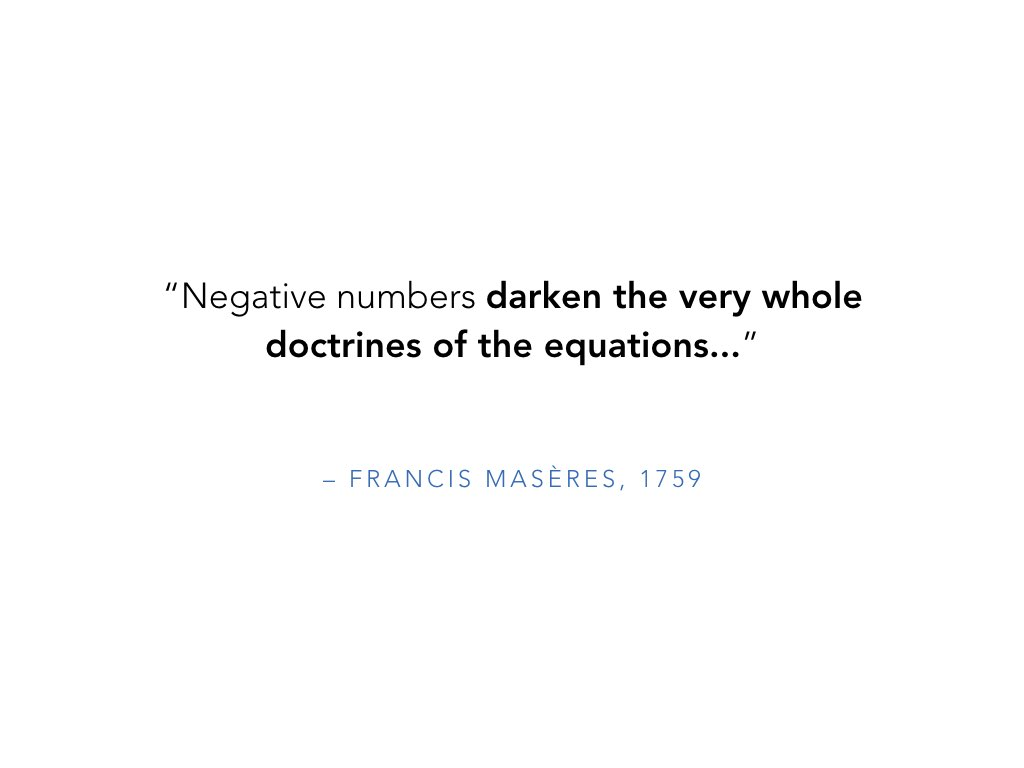In fact, even in 1759 people thought negative numbers were confusing. They "darken the very whole doctrines of the equations", right? They just didn't make sense. It was a weird concept and the problem was our analogy just couldn't handle it.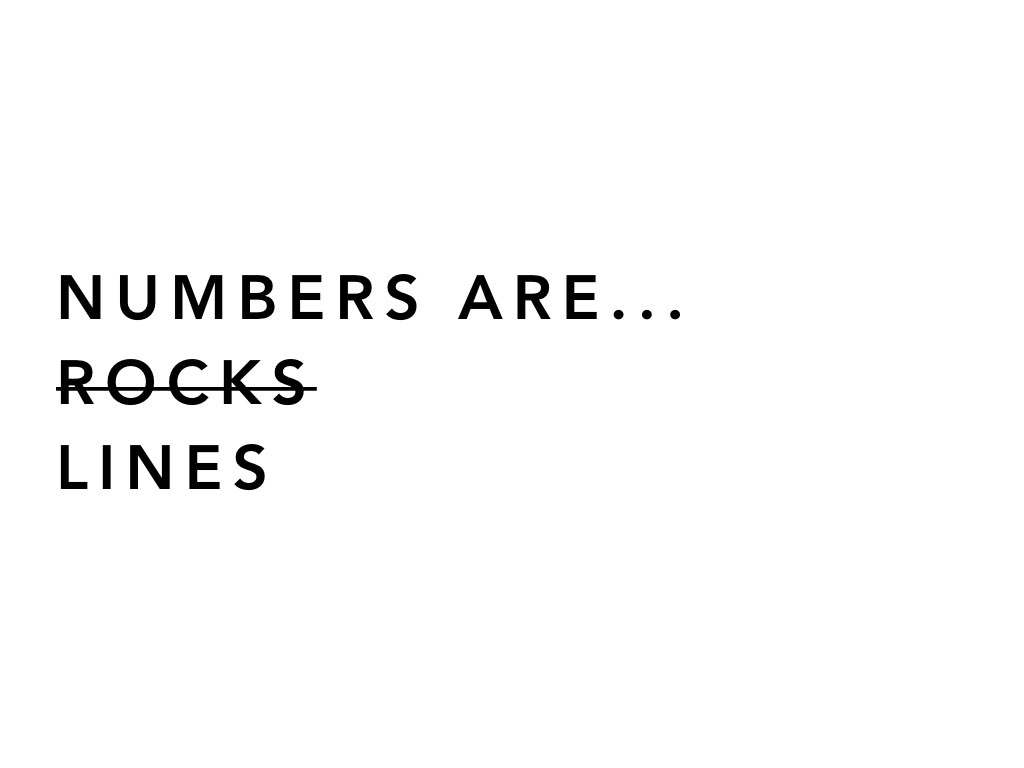But of course, any modern third grader can tell you we have the concept of a number line. Moving our mental model to a number line let negatives and zero be part of the picture.

Instead of zero as a void, it became the neutral point, the center, the place that every other number moves away from. That's a really cool concept -- positive and negative numbers aren't that different. They're left and right directions away from the same starting point.

Something that was really, really difficult became easy with the right analogy. Let's keep going.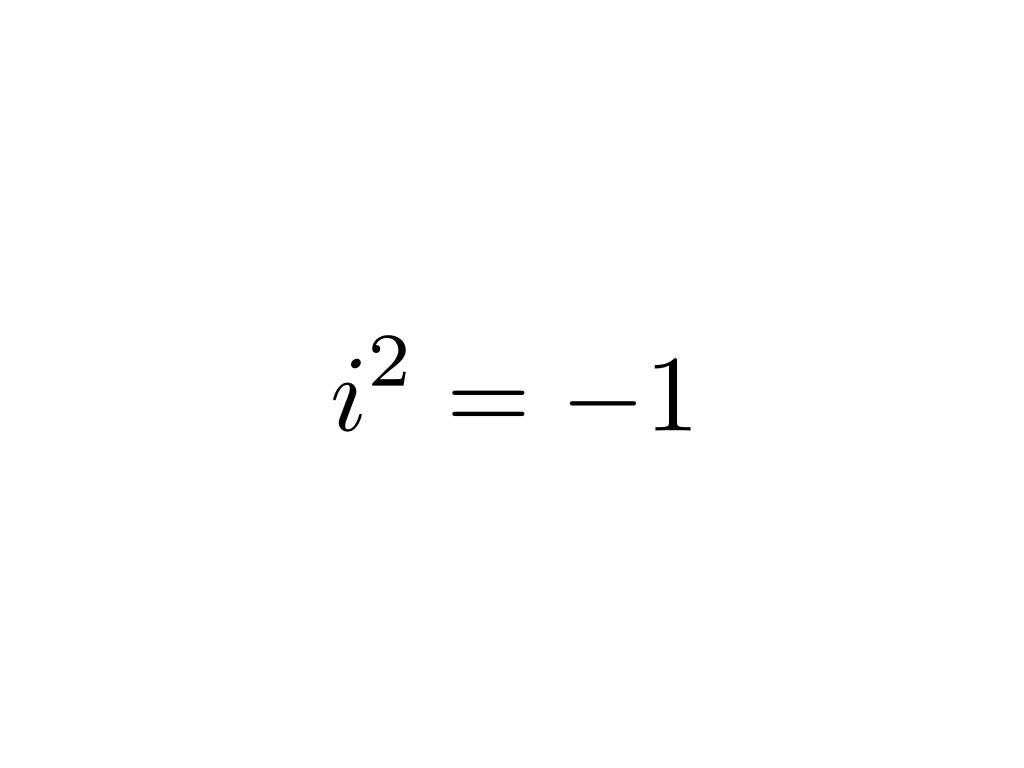How about something like this? i squared equals -1. This is super confusing. Our raft is starting to shake.

Is there any number that when you square it becomes a negative? Zero squared is still zero. A positive number, when squared, is still positive. A number number, when squared, becomes positive.

So, it seems like there's no solution. But again, this is like trying to find that negative rock. We're trying to apply the analogy in a way that limits our thinking. The analogy isn't the ultimate truth, right?

There might be a different way of thinking that makes it make sense, but we're stuck in this old system.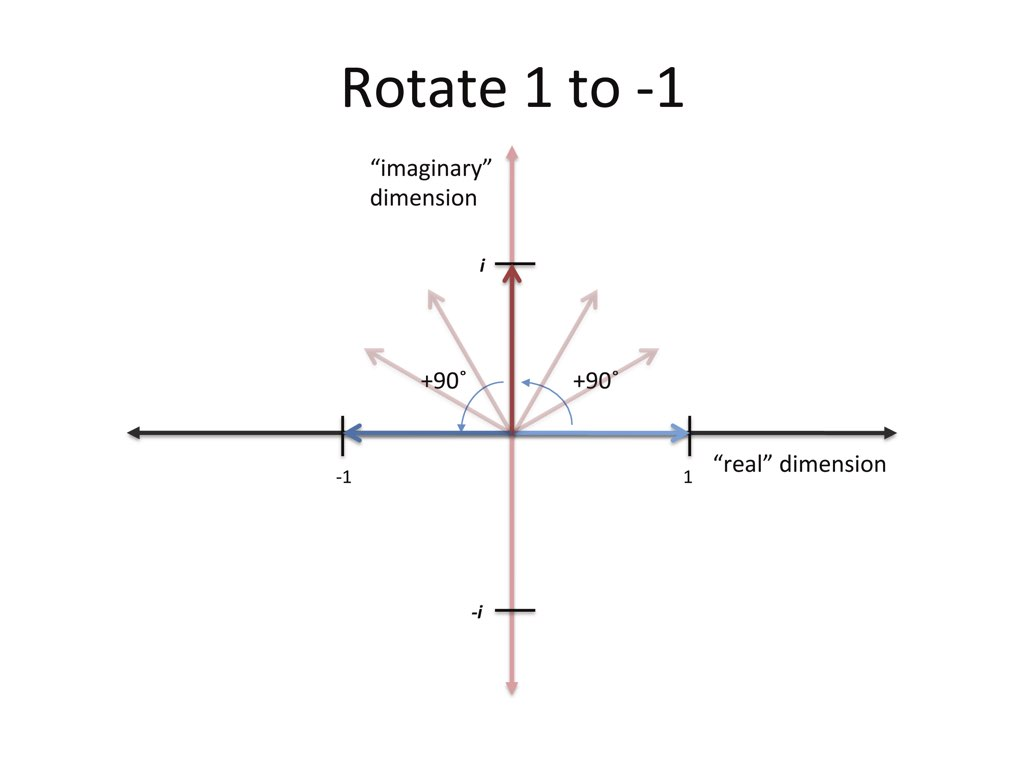Here's an idea: If we have a number line going left and right, why not another number line going north and south?

We have East/West, why not North/South too? Let's allow this extra dimension where numbers can move up and down, left or right.

The imaginary dimension lets us get to negatives in two steps. Saying "i squared is negative one" is really saying: I'm starting at 1. We multiply by i, multiply by i again, and get to -1. So i represents a 90-degree rotation, and rotating twice points you backwards. That's what "i squared equals -1" means.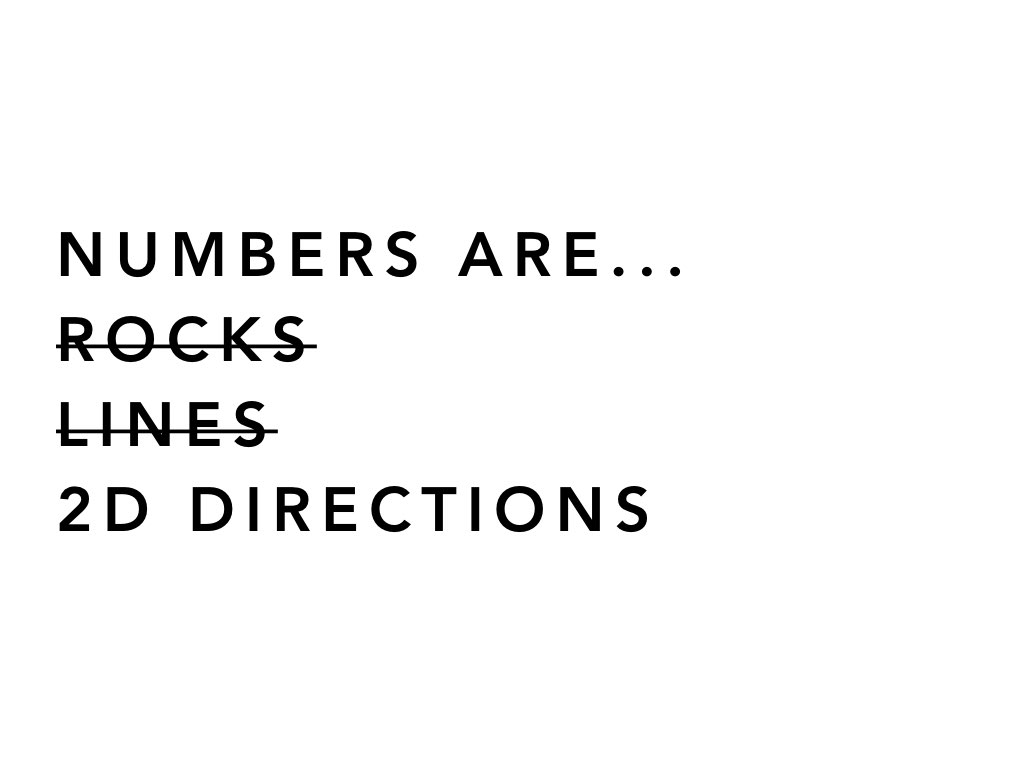Finding the right analogy makes something that seems baffling click, all because we're using the right metaphor. We're thinking about it the right way. So, we've gone from rocks to lines to directions and that seems pretty good.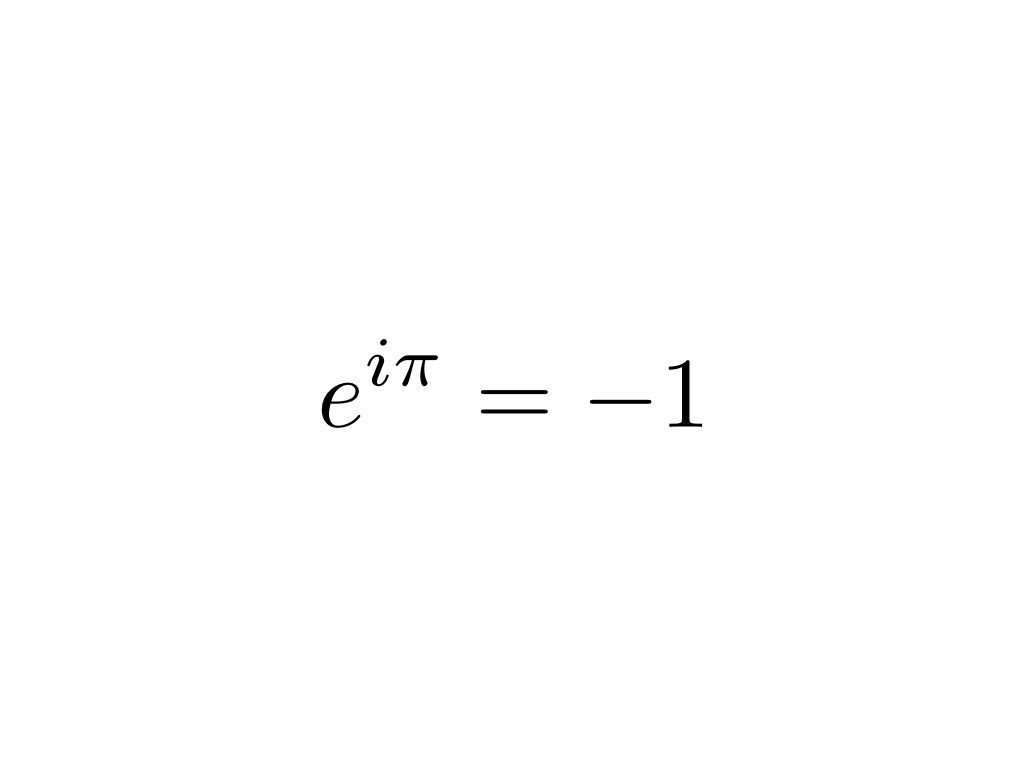Let's put this into action a little bit. Here's a very famous equation. Euler's Identity is one of the most beautiful in math.

If you ask a mathematician or a physicist, this is usually considered the most beautiful identity in all of mathematics. It relates to so many different concepts.The problem is it's really confusing and again, mathematicians thought it was just crazy when it came out. e to the i times pi equals negative one. We're taking these crazy constants: pi is irrational. i is this weird dimension, and e is another irrational number. All of these combined to equal -1 in such a clean way. It's wild.

And so people thought that OK, there are proofs, but it can't be understood. Again, that's coming from out existing analogies. If we have poor analogies for what numbers are, then yes, concepts like this are difficult, but with the right analogies they can make sense.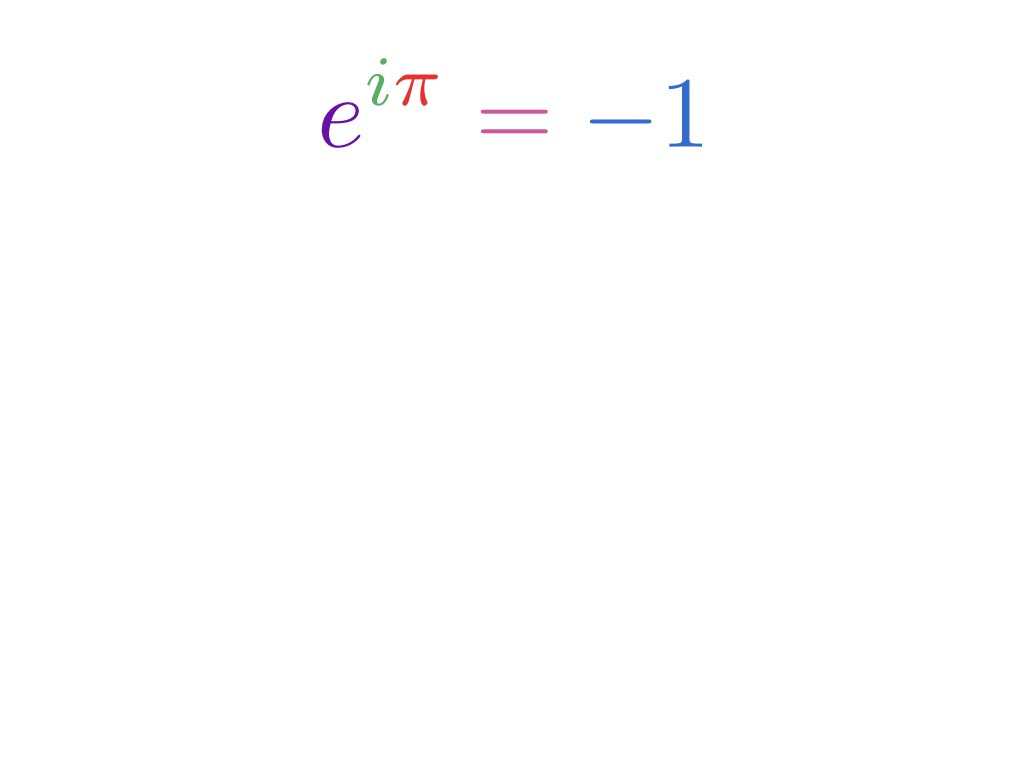So let's take a crack at it. I like to mentally colorize equations. I want to understand what every single part of the equation is trying to say.

Normally we look at equations as a mix of symbols, but if we can identify what each part is doing, it becomes a sentence. It becomes a story of what's actually happening. So let's start.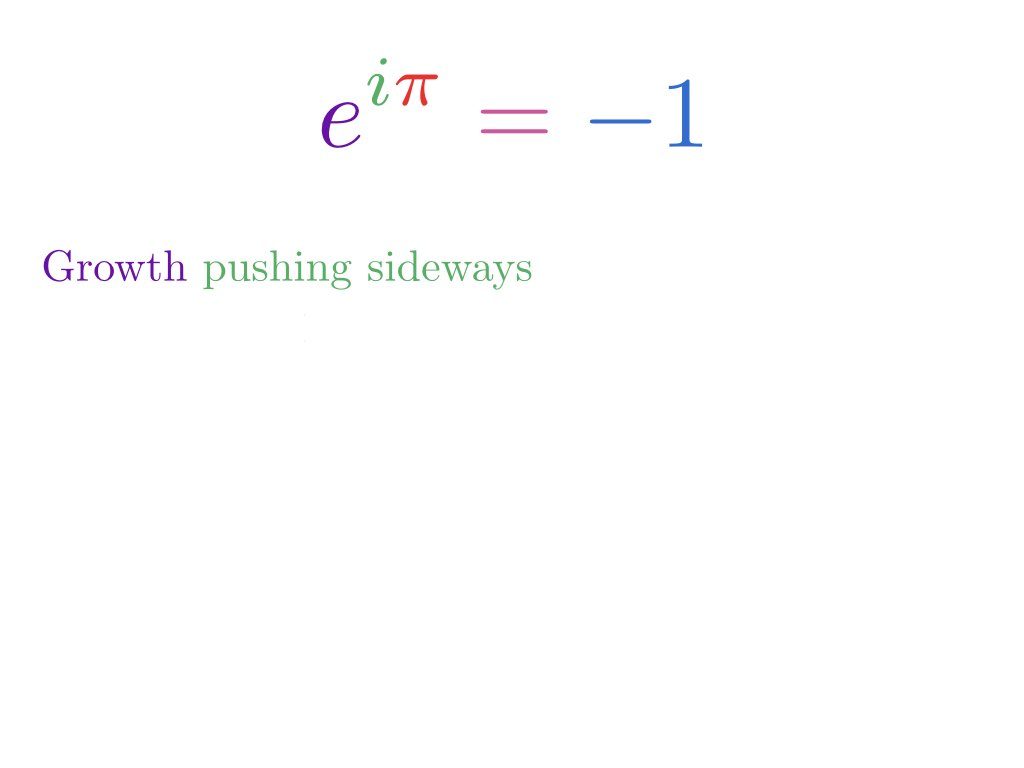e represents growth. It could be an entire separate video, but e is the concept of continuous growth, and i is the concept of going sideways (not the normal direction).

So the concept is that we have growth, but instead of growing like we want, we're growing sideways. And how long dow we do this for?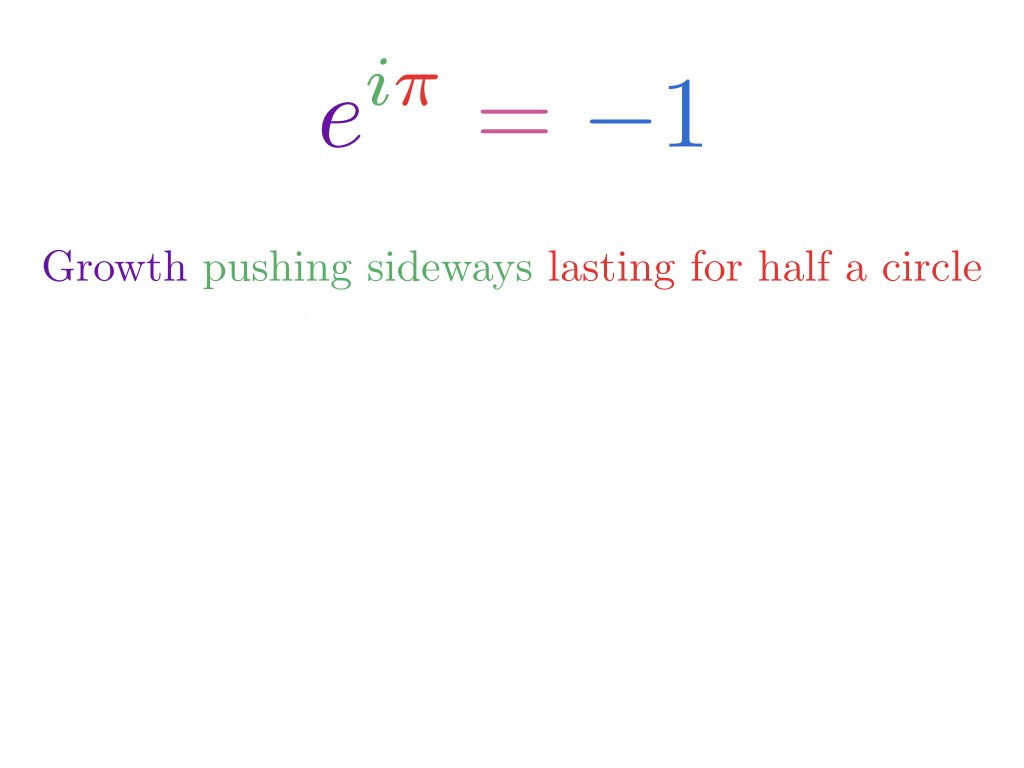Pi is halfway around a circle. A unit circle has two pi as its full circumference. So a single pi is just halfway around the circle.

We have the concept of growth going sideways that is lasting for half a circle. And if we plot it out, it looks like this: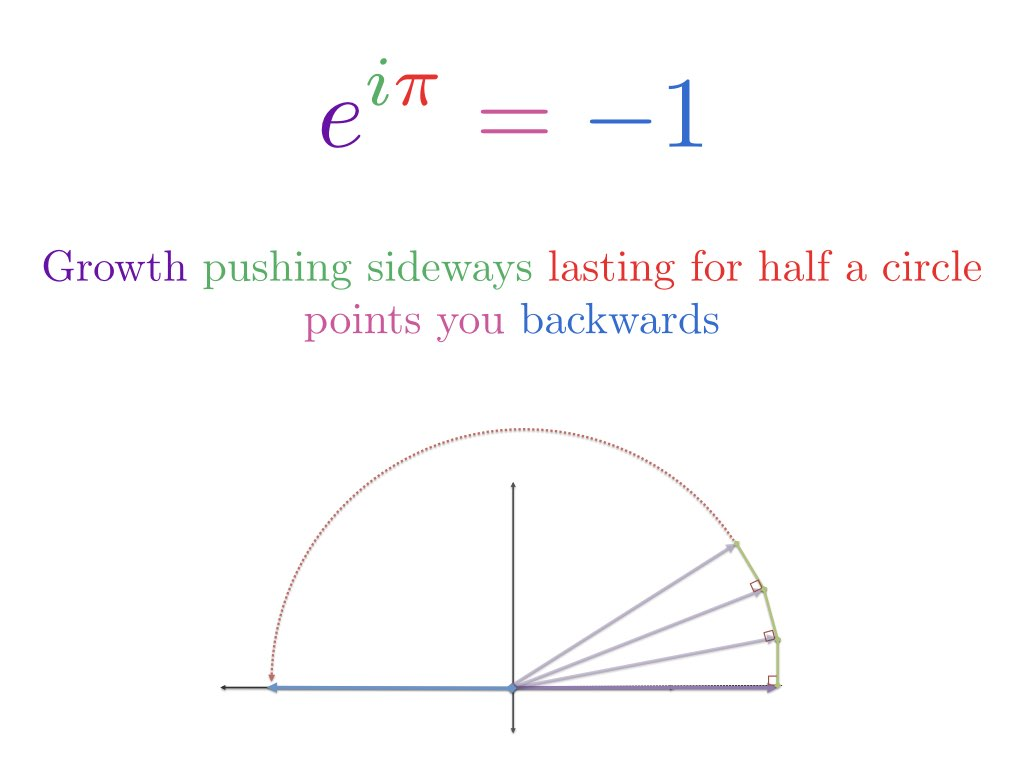We have growth, but it's going sideways, and we have enough fuel in the growth engine to point us backwards. So the equation is saying that this system of growth, pushing sideways, with enough fuel to last for half a circle, will point you backwards to negative one.

1 was the implied starting point. Start growing sideways with enough fuel to go half a circle and you'll end up backwards. So the key is we understood a concept that was baffling using a very simple diagram and analogy.

Now to really test it, let's try a few scenarios.

What it we don't put in any fuel?

What if we want to grow sideways, but we decided not to grow it all. We have the engine ready to go, but we don't fuel it up. If we put zero here instead, then we would stay at the starting point of 1.

Mathematically, we can say anything to the zeroth power is 1, which is true, but it's more helpful to think "I have this engine setup but didn't put any fuel into it, I never used it." Then you'll stay at your starting point.

If I put in twice as much fuel (2 * pi), I should circle all the way around. And again, we can mathematically say that if we square both sides, the result should be 1, but I like to think of it as using twice the fuel and going all the way around.This is how to take an analogy and put it to work, helping you understand.

The main takeaway for me is that good analogies make math a joy. An idea that's baffling can become simple, even enjoyable, if we see it the right way. That's the heart of my philosophy. If I'm confused by something, I don't think my CPU isn't good enough. I just think I had the wrong analogy.

Happy math.